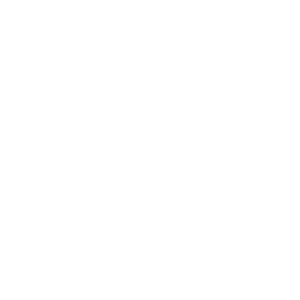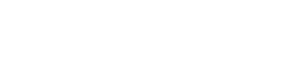0
247

# পদের লিখিত পরীক্ষার প্রশ্ন সমাধান-২০১৮

Bangladesh Development Bank Ltd. (BDBL) Written Questions Solution 2018

Post: Senior Officer

#### আরো পড়ুনঃ- বাংলাদেশ ডেভেলপমেন্ট ব্যাংক লিমিটেড সিনিয়র অফিসার পদের  প্রশ্ন সমাধান-২০১৭

BDBL Senior Officer Written Math (06-01-2018

A) The profit of a company is given in Taka by P = 3x²-35x+50, where x is the amount in Taka spent on advertising. For what values of x does the company make a profit?

Here given,

3x² – 35x + 50 > 0

=> 3x² – 30x – 5x + 50 > 0

=> 3x(x-10) – 5(x-10) > 0

=> (x-10)(3x-5) > 0

Here, the Roots of the equation are: 10 and 5/3

Since, the in-equation is Greater than 0; i.e. positive

So, range of x < 5/3; Or x>10

Ans. x < 5/3; Or x>10

B) An amount of Tk. 7200 is spent to cover the floor of a room by carpet. An amount of Tk. 576 would be saved if the breadth were 3 meters less. What is the breadth of the room?

let, length = x

area = xy

AQ, xy = 7200

or, x = 7200/y…….(1)

3 m less new breadth = y – 3 m

new area = x(y-3)

AQ, x(y-3)=7200-576

=> y = 37.5

C) Find the three digit prime number whose sum of the digits is 11 and each digit representing a prime number. Justify your answer.

Sum of digit to be 11 of a 3-digit number and each digit being a prime number, the number is: 227 or 353

In case of 227, sum of the digits is 2+2+7 = 11.

And 2, 2, 7 all the digits are prime.

Similarly, In case of 353, sum of the digits is 3+5+3 = 11.

And 3, 5, 3 all the digits are prime.

D) If a/(q-r) = b/(r-p) = c/(p-q) then show that, a+b+c = pa+qb+rc

Let, a/(q-r) = b/(r-p) = c/(p-q) = k

So, a = k(q-r); b = k(r-p); and c = k(p-q)

Now, L.H.S. => a+b+c = k(q-r)+ k(r-p)+ k(p-q) = k(q-r+r-p+p-q) = k x 0 = 0

And, R.H.S. => pa+qb+rc = p*k(q-r)+ q*k(r-p)+ r*k(p-q) = kpq-kpr+kqr-kpq+kpr-kqr = 0

So, L.H.S. = R.H.S. (Showed)

E) Prove that a cyclic parallelogram must be a rectangle.

Opposite angles in a parallelogram are congruent (equal), while opposite angles in a cyclic quadrilateral are supplementary (add up to 180°). Congruent supplementary angles are right angles, so opposite angles in a cyclic parallelogram are right angles. Thus all four angles are right angles, and it’s a rectangle.

Let ABCD be the cyclic parallelogram with angles A and C being opposite angles.

A = C

And, A+C = 180°

since A = C

So, A+A = 180°

2A = 180°

A = 90°

if any one angle of parallelogram is 90°, the parallelogram is a rectangle.

Or,F) After travelling 108 km, a cyclist observed that he would have required 3 hrs less if he could have travelled at a speed 3 km/hr more. At what speed did he travel?

S*T = 108

Again, (S+3)(T-3) = 108

=> ST – 3S + 3T – 9 = ST

=> 3T – 3S = 9

=> T – S = 3

=> T = S+3

Now, S*(S+3) = 108

=> S² + 3S – 108 = 0

=> S² + 12S – 9S – 108 = 0

=> S(S+12) – 9(S+12) = 0

=> (S+12)(S-9) = 0

S = 9 (Ans.)

SHORTCUT: 108 = 9*12 = 12*9

D = S*T

So, S = 9 and T = 12

G) Solve: x/2 + 6/y = 9, x/3 + 2/y = 4

1. g) Detail solution…

x/2+6/y = 9

or,18y – xy = 12….(1)

x/3+2/y=4

or,12y – xy=6…(2)

(1) – (2)

18y – xy =12

12y – xy =6

………………..

y = 1

putting the value equation (2)

12*1 – x*1 = 6

or, x = 6

so, ( x, y) = (6,1)